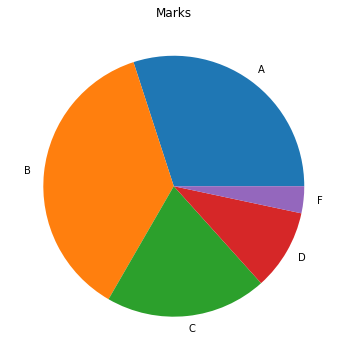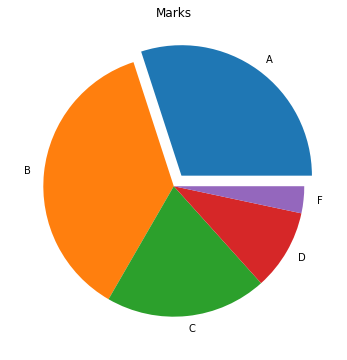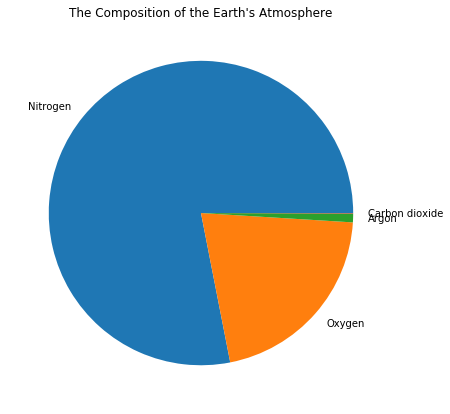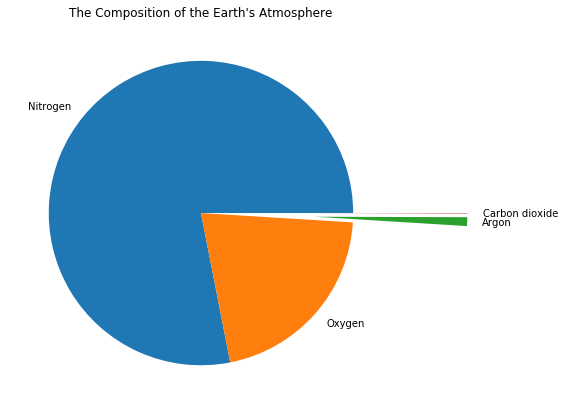# Obeleži sve kategorije koje odgovaraju problemu

### Još detalja - opišite nam problem

Uspešno ste prijavili problem!
Status problema i sve dodatne informacije možete pratiti klikom na link.
Nažalost nismo trenutno u mogućnosti da obradimo vaš zahtev.
Molimo vas da pokušate kasnije.

# 5. Frequency analysis and pie charts¶

In this lecture you will learn:

1. how to perform a frequency analysis of a sequence of data (which is just a fancy name for a simple thing); and
2. how to display data on a pie chart.

## 5.1. Frequency analysis¶

Frequency analysis of a sequence of values consists of counting how many times each value occurs in the sequence. As simple as that. The numbers that we get are called frequences. Nevertheless, this simple analysis can provide a significant insight into the problem we are interested in. It is even used in cryptoanalysis, but this is far beyond the scope of this handbok.

For example there are 30 students in a class and their Maths marks are:

In :
marks = ["C", "B", "A", "B", "A", "C", "B", "A", "D", "B", "A", "B", "A", "B", "D", "C", "F", "B", "A", "B", "C", "D", "C", "B", "A", "B", "A", "A", "B", "C"]


The frequency analysis of this sequence reduces to counting how many A's there are in the sequence, then how many B's, and so on. Instead of doing it by hand we'll let Python do it for us using the built-in function count:

In :
markA = marks.count("A")
markB = marks.count("B")
markC = marks.count("C")
markD = marks.count("D")
markF = marks.count("F")
print("The distribution of marks:")
print("A ->", markA)
print("B ->", markB)
print("C ->", markC)
print("D ->", markD)
print("F ->", markF)

The distribution of marks:
A -> 9
B -> 11
C -> 6
D -> 3
F -> 1


The numbers we have thus obtained are absolute frequencies. Often we are interested in relative frequencies, which are absolute frequences expressed as a percentage.

In :
N = len(marks)
percentageA = 100.0 * markA / N
percentageB = 100.0 * markB / N
percentageC = 100.0 * markC / N
percentageD = 100.0 * markD / N
percentageF = 100.0 * markF / N
print("The relative distribution of marks:")
print("A -> ", round(percentageA, 2), "%", sep="")
print("B -> ", round(percentageB, 2), "%", sep="")
print("C -> ", round(percentageC, 2), "%", sep="")
print("D -> ", round(percentageD, 2), "%", sep="")
print("F -> ", round(percentageF, 2), "%", sep="")

The relative distribution of marks:
A -> 30.0%
B -> 36.67%
C -> 20.0%
D -> 10.0%
F -> 3.33%


Therefore, the frequency analysis can provide absolute frequences (how many times does a value occur in the sequence), but also the relative frequences, which are absolute frequences expressed as percentages.

## 5.2. Pie charts¶

In situations where we are interested not so much in the absolute frequences, but more in the relative frequences (that is, we do not care much how many occurences there are, but what percentage that makes in the whole) it is convenient to visualize data as a pie chart. A pie chart is a circle divided into sectors (like a pie or a pizza). The circle then represents the whole (100%) and each sector represents the percentage of the value assigned to the sector.

For example, here is the distribution of the marks from the example above:

Ocena Zastupljenost ocene
A 9
B 11
C 6
D 3
F 1

Let us visualize this using a pie chart. Let us first load the library:

In :
import matplotlib.pyplot as plt


Then we represent the table as two lists:

In :
freqs = [9,   11,  6,   3,   1]
marks = ["A", "B", "C", "D", "F"]


Function pie produces a pie chart. The first argument of pie is a list of numbers (frequences, relative of absolute), while the option labels provides labels of sectors:

In :
plt.figure(figsize=(6,6))
plt.pie(freqs, labels=marks)
plt.title("Marks")
plt.show()
plt.close()If we wish to stress the number of A's in this class we can use the explode option which expects a sequence of decimal numbers between 0 and 1 which tells the pie function how much to slide the sector away from the center (0 = no sliding; the larger the number, the larger the sliding away from the center).

In :
freqs = [9,   11,  6,   3,   1]
marks = ["A", "B", "C", "D", "F"]
slide = [0.1,  0,   0,   0,   0]
plt.figure(figsize=(6,6))
plt.pie(freqs, labels=marks, explode=slide)
plt.title("Marks")
plt.show()
plt.close()As another example let us take a look at the structure of our atmosphere. Our atmosphere is a mixture of many gasses but the most dominant ones are:

Gas (%)
Nitrogen 78.08
Oxygen 20.94
Argon 0.93
Carbon dioxide 0.05

Here comes the pie chart:

In :
perc = [78.08,  20.94,      0.93,    0.05]
gas  = ["Nitrogen", "Oxygen", "Argon", "Carbon dioxide"]
plt.figure(figsize=(7,7))
plt.pie(perc, labels=gas)
plt.title("The Composition of the Earth's Atmosphere")
plt.show()
plt.close()We immediately see a problem: labels for the two tiny sectors overlap. To fix that we will slide them out using the explode option:

In :
perc  = [78.08,  20.94,      0.93,    0.05]
gas   = ["Nitrogen", "Oxygen", "Argon", "Carbon dioxide"]
slide = [0,      0,          0.75,    0.75]
plt.figure(figsize=(7,7))
plt.pie(perc, labels=gas, explode=slide)
plt.title("The Composition of the Earth's Atmosphere")
plt.show()
plt.close()## 5.3. Exercises¶

Exercise 1. Visualize the population of the continents by a pie chart:

Continent Population
Asia 4,584,807,072
Africa 1,320,038,716
Europe 743,102,600
S. America 658,305,557
N. America 366,496,802
Oceania 41,826,176

Exercise 2. Between 2006 and 2008 the market of optical storage media was a stage of the high definition optical disc format war. As HD became more and more popular, the need for a larger capacity optical disc emerged and the final battle for this market niche was fought between the Blu-ray Disc (BR) and HD-DVD. For more than two years the two formats had almost equal market share but then at the beginning of 2008 something strange happened -- within a week the situation changed drastically. The following table contains the market share of the two formats on January 5th, 2008 and seven days later:

Date Blu-ray Disc HD-DVD
January 5th, 2008 51,17% 48,83%
January 12th, 2008 92,53% 7,47%

So, after two years of bitter fighting Blu-ray won.

(a) Make two independent pie charts to visualize the market share of the two formats on January 5th, 2008 and on January 12th, 2008.

(b*) Search the Internet and try to find what happened in the week January 5-12th, 2008. (Hint: Has to do with Sony, who was a major proponent of Blu-ray Disc.)

Exercise 3. This is how you make the perfect lemonade: put a cup of warm water and a cup of sugar in a saucepan and stir until sugar dissolves completely; then add one cup of lemon juice and three cups of cold water.

Compute the percentage of water, sugar and lemon juice in the perfect lemonade and make a pie chart in which the sugar is slightly separated from the other ingredients.

Exercise 4. Watching it rain in Macondo, Isabel decided to start a meteorogical diary. If it rained on Monday Isabel would write 1 into the diary; if it rained on Tuesday she would write 2 into the diary; then 3 for rainy Wednesdays and so on until 7 for rainy Sundays. In the end she got the following list of numbers for a year:

In :
MeteoDiary = [1,2,4,7,2,4,7,6,7,5,6,7,3,5,7,1,3,6,2,3,4,2,3,1,4,7,7,
6,5,6,4,5,6,2,3,4,5,1,3,4,2,5,7,2,3,5,3,5,7,6,7,2,3,7,
1,2,3,4,5,6,7,2,7,3,4,1,5,6,1,2,4,5,6,7,1,3,4,1,2,3,4,
2,5,7,6,4,5,6,1,3,7,5,7,1,2,3,7,7,3,4,7,1,2,4,7,4,7,2,
3,4,4,6,8,1,7,7,7,3,4,5,6,7,1,2,4,7,1,2,3,1,7,2,7]


(a) How many rainy days did Isabel record?

(b) How many Mondays, Tuesdays and so on were there that year? Illustrate this by a pie chart.

(c) Which day of the week was the rainiest?

Exercise 5. The cell below contains the first few decimals of $\pi$:

In :
pi_decimale="141592653589793238462643383279502884197169399375105820974944592307816406286208998628034825342117067982148086513282306647093844609550582231725359408128481117450284102701938521105559644622948954930381964428810975665933446128475648233786783165271201909145648566923460348610454326648213393607260249141273724587006606315588174881520920962829254091715364367892590360011330530548820466521384146951941511609433057270365759591953092186117381932611793105118548074462379962749567351885752724891227938183011949129833673362440656643086021394946395224737190702179860943702770539217176293176752384674818467669405132000568127145263560827785771342757789609173637178721468440901224953430146549585371050792279689258923542019956112129021960864034418159813629774771309960518707211349999998372978049951059731732816096318595024459455346908302642522308253344685035261931188171010003137838752886587533208381420617177669147303598253490428755468731159562863882353787593751957781857780532171226806613001927876611195909216420198938095257201065485863278865936153381827968230301952035301852968995773622599413891249721775283479131515574857242454150695950829533116861727855889075098381754637464939319255060400927701671139009848824012858361603563707660104710181942955596198946767837449448255379774726847104047534646208046684259069491293313677028989152104752162056966024058038150193511253382430035587640247496473263914199272604269922796782354781636009341721641219924586315030286182974555706749838505494588586926995690927210797509302955321165344987202755960236480665499119881834797753566369807426542527862551818417574672890977772793800081647060016145249192173217214772350141441973568548161361157352552133475741849468438523323907394143334547762416862518983569485562099219222184272550254256887671790494601653466804988627232791786085784383827967976681454100953883786360950680064225125205117392984896084128488626945604241965285022210661186306744278622039194945047123713786960956364371917287467764657573962413890865832645995813390478027590099465764078951269468398352595709825822620522489407726719478268482601476990902640136394437455305068203496252451749399651431429809190659250937221696461515709858387410597885959772975498930161753928468138268683868942774155991855925245953959431049972524680845987273644695848653836736222626099124608051243884390451244136549762780797715691435997700129616089441694868555848406353422072225828488648158456028506016842739452267467678895252138522549954666727823986456596116354886230577456498035593634568174324112515076069479451096596094025228879710893145669136867228748940560101503308617928680920874760917824938589009714909675985261365549781893129784821682998948722658804857564014270477555132379641451523746234364542858444795265867821051141354735739523113427166102135969536231442952484937187110145765403590279934403742007310578539062198387447808478489683321445713868751943506430218453191048481005370614680674919278191197939952061419663428754440643745123718192179998391015919561814675142691239748940907186494231961567945208095146550225231603881930142093762137855956638937787083039069792077346722182562599661501421503068038447734549202605414665925201497442850732518666002132434088190710486331734649651453905796268561005508106658796998163574736384052571459102897064140110971206280439039759515677157700420337869936007230558763176359421873125147120532928191826186125867321579198414848829164470609575270695722091756711672291098169091528017350671274858322287183520935396572512108357915136988209144421006751033467110314126711136990865851639831501970165151168517143765761835155650884909989859982387345528331635507647918535893226185489632132933089857064204675259070915481416549859461637180270981994309924488957571282890592323326097299712084433573265489382391193259746366730583604142813883032038249037589852437441702913276561809377344403070746921120191302033038019762110110044929321516084244485963766983895228684783123552658213144957685726243344189303968642624341077322697802807318915441101044682325271620105265227211166039666557309254711055785376346682065310989652691862056476931"


(a) How many decimals of $\pi$ are there in the string?

(b) Make the frequency analysis of this string.

(g) Illustrate the outcome of the frequency analysis by a pie chart.